HPS 0410 Einstein for Everyone

Back to main course page

Special Theory of Relativity: The Principles

John D. Norton
Department of History and Philosophy of Science
University of Pittsburgh

Background reading: J. Schwartz and M. McGuinness, Einstein for Beginners. New York: Pantheon.. pp. 66 - 151.

"On the Electrodynamics of Moving Bodies"

In June 1905, when Albert Einstein was still a patent examiner in Bern, Switzerland, he sent a paper with this title to the journal Annalen der Physik. It contained his special theory of relativity. He argued that altering our understanding of the behavior of space and time could resolve certain problems in electrodynamics. (See page one in German or English.)

The theory Einstein described is now known as the "special theory of relativity." It is a "theory of relativity" because it is based on the relativity of inertial motion. The qualification "special" was not originally part of the theory. Over the coming decade, Einstein sought to develop his theory of 1905 in a way that would extend the treatment of relative motion to accelerated motion and would, at the same time, incorporate gravitation. The theory of 1905 came to be called the "special" theory to distinguish it from the later extension, "the general theory of relativity."

To understand Einstein's special theory, we need some preliminary notions.

## Inertial and Accelerated Motion

 There is a preferred motion in space known as inertial motion. Any body left to itself in space will default to an inertial motion, which is just motion at uniform speed in a straight line. The easiest example to visualize is a huge spaceship with the engines turned off, gliding through some remote part of space, far away from stars and planets. At any point in space, many inertial motions are possible. They will be pointed in different directions and will be at different speeds. The existence of such motions as the natural default is a fundamental fact about space and time. We need to discover which of all possible motions in space comprise the inertial motions. We do that by observing things in motion when they are free of deflecting forces; or by correcting the motions we observed for the deflecting effects of these motions. This is an important point. The loose talk surrounding relativity theory could lead one to think that empty space is nothing at all. That idea is not sustainable in special relativity. In the theory, empty space is rich in structure. Part of that structure is the existence of special motions, the inertial motions.Based on a file that has been donated to the Wikimedia Foundation and released into the public domain by Pearson Scott Foresman.http://commons.wikimedia.org/wiki/File:Spacecraft_(PSF).pngAny other motion is accelerated. This includes motion at uniform speed in a circle. While the speed stays the same, the direction does not. So the motion is accelerated.
 Sometimes we will talk of an "inertial observer." It is just an observer who moves inertially.Such an observer might set up an elaborate system of measuring rods and other physical devices to fix the positions of events; and an elaborate system of clocks to fix their timing. Such a system is an inertial frame of reference. The figure shows one way that we might build an inertial frame. We fill space with an array of clocks and use them to determine the time of an event coincident with the clock. We connect the clocks with measuring rods so that we can locate any point in the frame merely by giving directions in the form of three numbers. "(4,3,7)" means, say, go right 4 rods from the starting point, up 3 and then back 7. We identify different events at the spatial location in the frame by the different times read by the clock at that location. What is important is that the frame consists of physical devices--typically measuring rods and clocks--that are at rest relative to the inertial observer. This is important since we shall see shortly that the lengths and times read by such devices are affected by their motion, according to special relativity.
 We measure times elapsed with these clocks and distances with these rods. That is, when lengths, times and other quantities are referred to an inertial frame, we speak of the magnitudes that would result from measurements using the rods and clocks of the inertial frame. If an asteroid fragment is "100 feet long in the inertial frame," that means that it would be measured to have a length of 100 feet by the measuring rods of the frame. If an astronaut taps a finger on the controls of a spaceship "once per second in the inertial frame," those seconds are measured by the clocks of the inertial frame.It is the same when we speak of motion with respect to an inertial frame of reference. If some spaceship moves uniformly in a straight line in the frame, that means that it covers equal distances in the frame in equal time, while moving in one direction. The "equal distances" are distances as measured by the rods of the frame. The equal times are times as measured by the frame's clocks. Such a motion is shown in the figure. The spaceship passes from clock to clock in equal times, as indicated by the clocks recording "1," "2,", "3," etc. when it passes them. The distances between the clocks are the same: one rod length. Therefore the spaceships moves equal distances in equal times (and in the same direction.)
 Strictly speaking, an inertial frame must be realized by introducing real rods and clocks into space. The above scheme would require that space be filled by them. That is not the sort of thing that can be done easily. In practice, it is rarely done. For we can predict in most cases what measurements would result were we to fill space with rods and clocks of an inertial frame of reference. When we say the spaceship is "moving uniformly in a straight line in the inertial frame," we really mean that if we were to fill the space with the appropriate rods and clocks, we would measure it to be moving so.## Absolute versus Relative Motion

 Relative motion arises when one body moves with respect to another. For example, our spaceship might move relatively to a nearby planet.Correspondingly the planet moves relative to the spaceship. These are two ways of describing the same relative motion.

See an animation.

Prior to Einstein, it was generally thought that there was another sense of motion, absolute motion. According to this sense, there is a fact of the matter as to whether the spaceship is moving, without regard to whether it moves relative to another object, such as a planet. There is an absolute state of rest in space, according to this earlier view. Either the spaceship is in this state and at rest; or it is not and it is moving. Einstein's theory did away with this notion of absolute rest and absolute motion.

Under this earlier, discarded view, this spaceship just moves, period. That it moves does not require any further reference to other objects. It is an unconditional, that is, absolute, fact.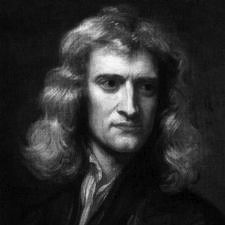The terms "absolute motion," "absolute place and "absolute space" were introduced by Isaac Newton as part of the foundational work of his Principia. There he laid out the a system of mechanics that we now know as Newtonian. In his introductory discussion, he wrote: "Absolute motion is the translation of a body from one absolute place into another; and relative motion, the translation from one relative place into another." Einstein's theory of relativity is usually understood as a repudiation of these notions of Newton's.

We shall see that Einstein's theory does discard the absolutes of Newton's physics by introducing the idea of the relativity of all inertial motion. That means that we cannot distinguish any special motions among all the inertial motions, such as a preferred rest state. We cannot say that this one is fast, that one is slow and another is at rest. We must always add a qualification. We must say that this is one is fast with respect to that; that one is slow with respect to another; or at rest with respect to another again.

In his special theory of relativity, the relativity of motion is implemented only for inertial motions. It does not extend to accelerated motions. They are motions that change their speed or direction or both. We can still say that something accelerates without adding a further qualification "with respect to...". Thus, acceleration is an absolute for Einstein's special theory.

Acceleration is discoverable by an experiment within a closed body. If the body is accelerating, that will be revealed by effects within the body. For example, an airplane flies inertially until it hits an air pocket. Then the plane lurches--accelerates--and things inside are thrown about. Everyone inside has no doubt of the acceleration.

Beware of oversimplified slogans. slogan like "It's all relative." or "All motion is relative." That is not what we learn from Einstein's special theory. It eradicates absolute motion. We learn that something can only be at rest if it is at rest with respect to something else. So the better slogan would be "All rest is relative."

Einstein found it most convenient to base his theory of relativity on two postulates; once they were assumed it became an exercise in logic to develop the whole theory. The two postulates are
I. The Principle of Relativity and
II. The Light Postulate.

## I. The Principle of Relativity

The first principle, the principle of relativity, says something about all the laws of physics. To state the principle, we must first express those laws in a particular way, that is, in terms of the quantities defined within an inertial frame of reference.

Take Newton's first law of motion, for example. (It is a law that also holds in special relativity.) It says in Newton's original wording:

Every body continues in its state of rest, or in uniform motion in a right [straight] line, unless it is compelled to change that state by forces impressed upon it.

The terms used in this formulation of Newton's first law require that we refer to some inertial frame of reference. "State of rest" means that the body remains at the same position in the inertial frame over time. "Uniform motion" means that the body covers the same distance in the same time, where distance is measured by the frame's measuring rods and time is measured by the frame's clock.

The principle of relativity asserts:

The laws of physics are the same in all inertial frames of reference.

That just means this. If we express some law of physics using the quantities of one inertial frame of reference, the resulting statement of the law will be exactly the same in any other inertial frame of reference.

We can use the example of Newton's first law of motion to illustrate the principle. Assume that we have three different inertial frames of reference, corresponding to the three observers shown below, then the statement of the law is the same in each frame. That means that we write down exactly the same sentence to express the law in each inertial frame. The terms like "state of rest" and "uniform motion" are to be expressed in the quantities of each frame.In this inertial frame of reference, Newton's first law of motion reads: Every body continues in its state of rest, or in uniform motion in a right [straight] line, unless it is compelled to change that state by forces impressed upon it. In this inertial frame of reference, Newton's first law of motion reads: Every body continues in its state of rest, or in uniform motion in a right [straight] line, unless it is compelled to change that state by forces impressed upon it. In this inertial frame of reference, Newton's first law of motion reads: Every body continues in its state of rest, or in uniform motion in a right [straight] line, unless it is compelled to change that state by forces impressed upon it.

That the law has exactly the same form in each inertial frame of reference precludes absolute motions.

To see why absolute motions are precluded, consider a pre-Newtonian view of motion. According to it, all motions would automatically slow down until they arrived at a unique state of rest. This law cannot be formulated in the same way in all inertial frames of reference. We would need one form of the law if the frame at issue is the true rest frame. We would need a different form in all the other frames of reference.

This is illustrated below. Assume that the middle motion is the unique state of rest "A". Then the pre-Newtonian law is expressed differently in the other two frames and in a way that requires specific mention of the unique state of rest "A".The unique state of rest "A".In this frame of reference, the pre-Newtonian law reads: Every body alters its motion until it moves like "A". In this frame of reference, the pre-Newtonian law reads: Every moving body slows to rest. In this frame of reference, the pre-Newtonian law reads: Every body alters its motion until it moves like "A".

That is, we infer from the principle of relativity:

Absolute motion cannot appear in any law of physics.

Note: we infer this from the principle of relativity. So this conclusion might not say as much as the principle does. It might be logically weaker. That is, this conclusion is not the principle itself.

There are more important conclusions that we will draw from the principle of relativity. One of the most useful pertains to experiments. To make the connection, we need to see that laws of nature are really compact summaries of a huge range of possibilities.

For example, Ohm's law just tells us that the voltage across a resistor is proportional to the current flowing. That proportionality is really a summary statement of a huge number of facts, each of which applies to a specific case.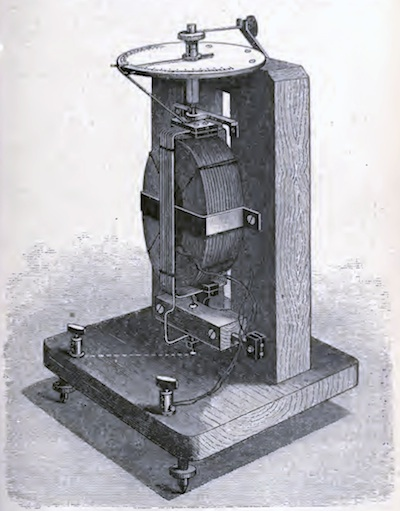Siemens electro-dynamometer for measuring strong currents. p.58 in J. E. H. Gordon, School Electricity. London: Sampson Low, Marston, Searle, & Rivington, 1886. It says: For a 1 Ohm resistor: When the voltage is 1V, the current is 1A. When the voltage is 2V, the current is 2A. When the voltage is 3V, the current is 3A. When the voltage is 4V, the current is 4A. ... For a 2 Ohm resistor: When the voltage is 2V, the current is 1A. When the voltage is 4V, the current is 2A. When the voltage is 6V, the current is 3A. When the voltage is 8V, the current is 4A. ... And so on indefinitely for many more cases.

Each one of these possibilities describes a little experiment and tells us what the result must be. For example, one of them tells us that, if we procure a 1 Ohm resistor and apply a voltage of 2V ("Volts") to it, 2A ("Amps") of current will flow.

Imagine now that we carry out this experiment in one inertial frame of reference. Assume that the experiment succeeds, returning the above result of 2A of current. We now know that this possibility is one of the possibilities listed by the applicable law of physics.

Since the laws are the same in each inertial frame of reference, it follows that the lists of possibilities in each inertial frame of reference are the same. Hence if the experiment proceeds as described in one inertial frame of reference it must also proceed in the same way in any other inertial frame of reference.

Combining these last two ideas we conclude:

All experiments run the same in all inertial frames of reference.

We can represent this consequence using the three inertial frame of reference described earlier, as follows:A voltage of 2V was applied to a 1 Ohm resistor. 2A of current flowed. A voltage of 2V was applied to a 1 Ohm resistor. 2A of current flowed. A voltage of 2V was applied to a 1 Ohm resistor. 2A of current flowed.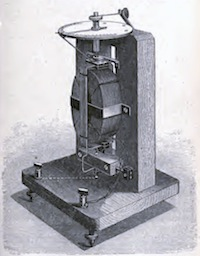If we turn this result around, we can recover another useful consequence of the principle of relativity. All experiments run the same in all inertial frames of reference. That means that anything that happens in one inertial frame can also happen in any other. It follows that there can be no sign that the inertial frame you are in is intrinsically special, that is, in itself different from the others. Thus no experiment can pick out just one inertial frame of reference as distinguished. Since absolute rest and absolute motion, if detectible, would require just such a distinguished rest frame, we can conclude: What an experiment can do it to determine whether the inertial frame is moving with respect to something else. That does not show there is anything intrinsically special about the inertial frame. For example, if a spaceship is near enough to some distant star for it to be visible, the astronaut merely needs to look out the window to see whether there is relative motion with respect to that star. There will be only one inertial frame for the spaceship in which the star will be judged to be at rest. In this sense, an experiment--observing the star--can distinguish a single preferred frame. However it is not the sense of distinguished that would violate the principle of relativity. For what the observation of the star shows is an accidental property of the inertial system, not one that is intrinsic to it. It is akin to the accidental fact that the spaceship is at rest in that inertial frame. That does not distinguish the inertial frame as intrinsically different from the others. The spaceship can readily be moved to any other inertial frame merely by means of a short blast from its rocket motors. Correspondingly, as far as special relativity is concerned, that star is not fixed in the inertial frame. It could in principle be set in motion so it comes to be at rest in another inertial frame of reference.

No experiment can reveal the absolute motion of the observer.

This is a consequence of the principle of relativity. Another way to see it is to recall that the principle of relativity leads us to conclude that absolute motion cannot figure in any law of physics. But if it is not in the laws and the laws determine what can be, then absolute motion cannot be. So no experiment could detect it!

Finally, to repeat a remark made earlier, we need to preclude a misunderstanding can arise easily. It is common to see loose talk that all motion is relative. This is careless talk. The principle of relativity of Einstein's special theory is restricted to inertial motion. The relativity it asserts does not extend to accelerated motion. If something accelerates, then it does so absolutely in Einstein's theory. There is no need to say that it "accelerates with respect to..." A traditional indicator of acceleration is inertial forces. If you are in an airplane that flies uniformly in a straight line, you have no sense of motion. If the airplane hits turbulence and accelerates, you sense immediately the acceleration. Inertial forces throw things around in the cabin. Those effects reveal the absolute acceleration of the airplane.Here is the principle of relativity and the three important consequences collected in one place.

Principle of Relativity: The laws of physics are the same in all inertial frames of reference.

It follows that:

Absolute motion cannot appear in any law of physics.
All experiments run the same in all inertial frames of reference.
No experiment can reveal the absolute motion of the observer.

Before we move on to the second principle, here's an animation that shows the key idea of the principle of relativity applied to experiments. Imagine two spaceships, each moving inertially in space but with different velocities. If we conduct the same experiments on each ship, we will end up with the same result ("27") no matter which spaceship we are on:## II. The Light Postulate

The light postulate is much narrower in scope. It makes no reference to all the laws of physics, as does the principle of relativity. Rather it refers to the propagation of light. If we have light propagating in any inertial frame, we measure its speed using the rods and clocks of that frame. We will find:

The speed of light is the same in all inertial frames of reference.

That speed is 186,000 miles per second or 300,000 kilometers per second. Because this speed crops up so often in relativity theory, it is represented by the letter "c".

The speed referred to here is the speed of light in a vacuum. Light passing through any dense medium, such as glass or water, is appreciably slowed by the medium. Light in water travels at 75% its speed in a vacuum. There is even a very slight effect in air. But it is very slight: a slowing of 0.03%That Einstein should believe the principle of relativity should not come as such a surprise. We are moving rapidly on planet earth through space. But our motion is nearly invisible to us, as the principle of relativity requires. Why nearly invisible? It is because the motion at any point on the earth's surface is not quite inertial. In the course of a full day, the direction of motion of the point will turn through a full 360 degrees. This very slow turning produces effects that can be measured, by, for example, the Foucault pendulum experiment that is so popular in science museums.

Why Einstein should believe the light postulate is a little harder to see. We would expect that a light signal would slow down relative to us if we chase after it. The light postulate says no. No matter how fast an inertial observer is traveling in pursuit of the light signal, that observer will always find the light signal to be traveling at the same speed, c.

c

The principal reason for Einstein's acceptance of the light postulate was his lengthy study of electrodynamics, the theory of electric and magnetic fields. The theory was the most advanced physics of the time. Some 50 years before, Maxwell had shown that light was merely a ripple propagating in an electromagnetic field. Maxwell's theory predicted that the speed of the ripple was a quite definite number: c .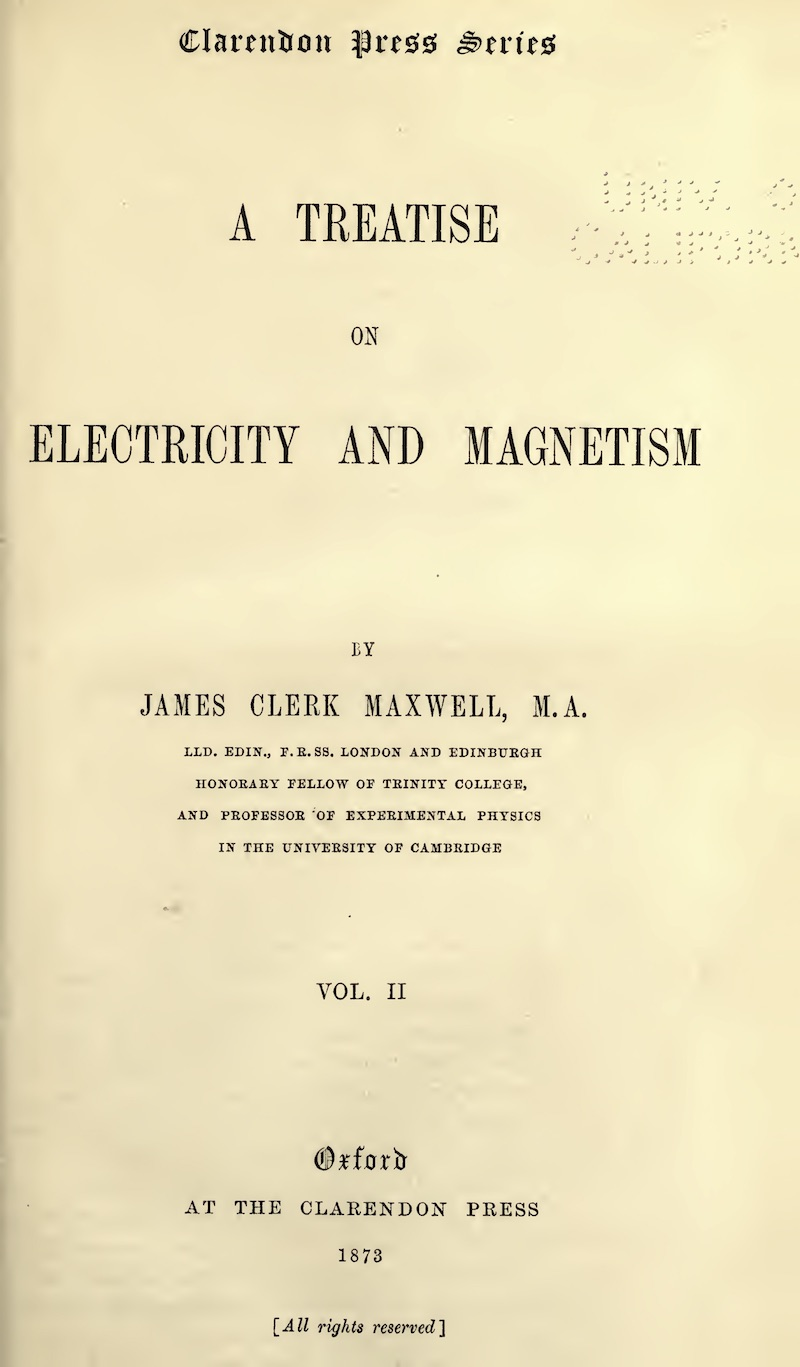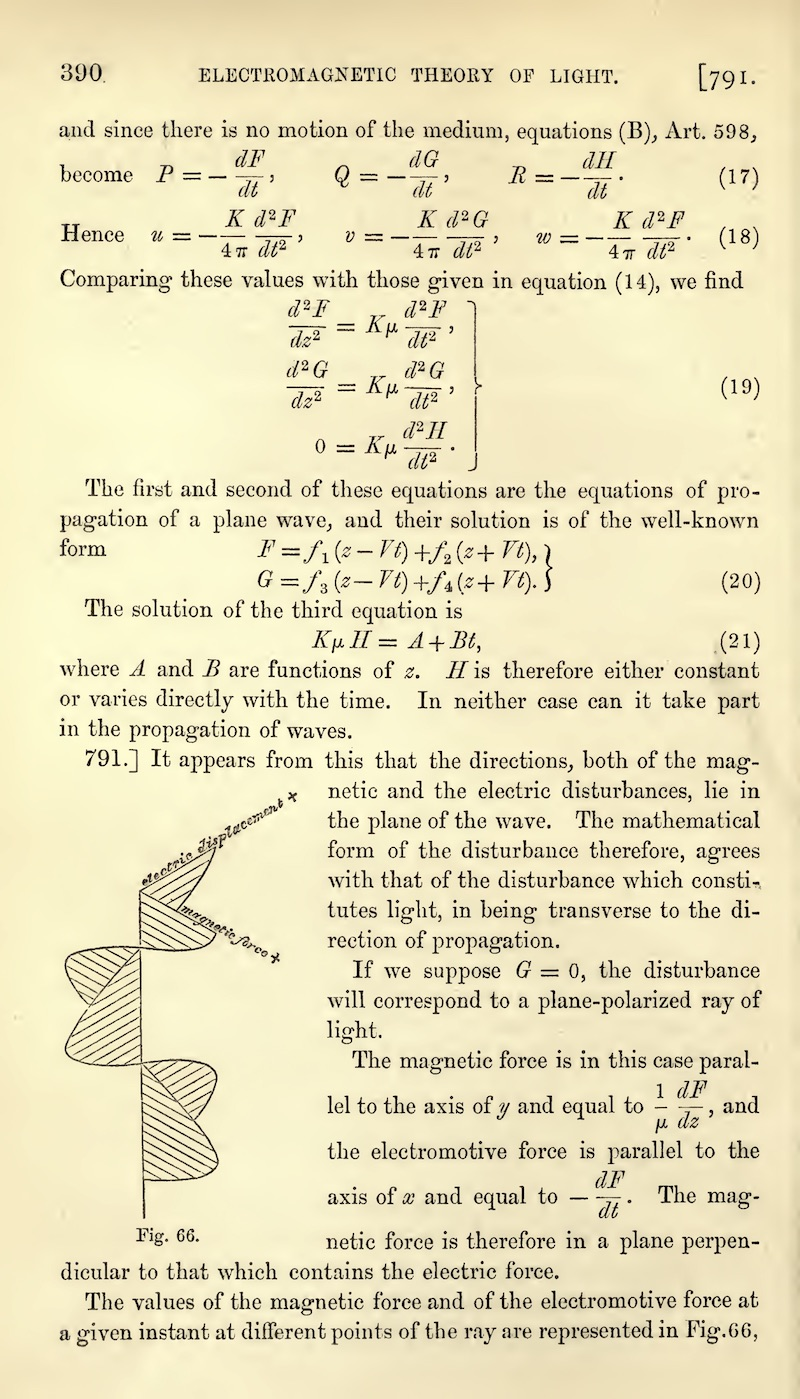The speed of a light signal was quite unlike the speed of a pebble, say. The pebble could move at any speed, depending on how hard it was thrown. It was different with light in Maxwell's theory. No matter how the light signal was made and projected, its speed always came out the same. For Maxwell, that speed was always the same in just one frame of reference, the rest frame of his electromagnetic ether.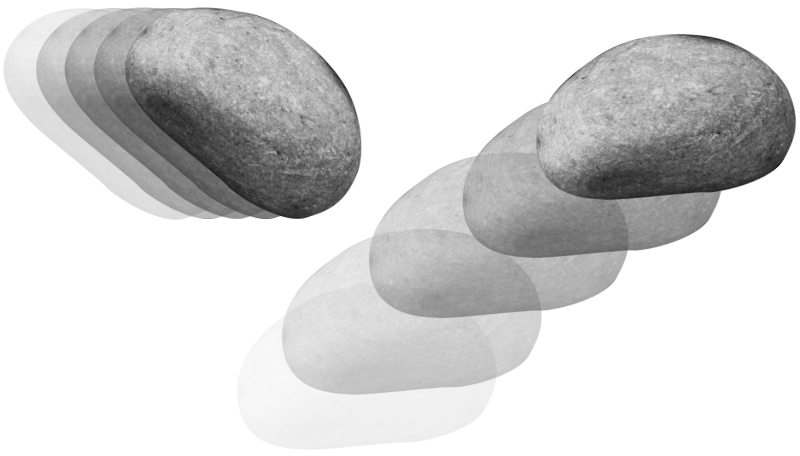The principle of relativity assured Einstein that the laws of nature were the same for all inertial observers. That light always propagated at the same speed was a law within Maxwell's theory. If it held in one inertial frame of reference, it must hold in all. So, if the principle of relativity was applied to Maxwell's law about light, the light postulate resulted immediately.

## What you need to know:

• Inertial and accelerated motion.
• Absolute versus relative motion.
• Inertial frames of reference.
• Einstein's two postulates and how to apply them.

Copyright John D. Norton. January 2001; August 30, 2002; July 20, 2006; January 8 2007; January 3, August 21, 27, 2008; January 6 10, 2011; February 10, 2013. January 14, 2022.